## 4.9 Static Expressions and Static Subtypes -- TOC

(1)
Certain expressions of a scalar or string type are defined to be static. Similarly, certain discrete ranges are defined to be static, and certain scalar and string subtypes are defined to be static subtypes. Static means determinable at compile time, using the declared properties or values of the program entities.
(2)
A static expression is a scalar or string expression that is one of the following:
(3)
• a numeric_literal;
(4)
• a string_literal of a static string subtype;
(5)
• a name that denotes the declaration of a named number or a static constant;
(6)
• a function_call whose function_name or function_prefix statically denotes a static function, and whose actual parameters, if any (whether given explicitly or by default), are all static expressions;
(7)
• an attribute_reference that denotes a scalar value, and whose prefix denotes a static scalar subtype;
(8)
• an attribute_reference whose prefix statically denotes a statically constrained array object or array subtype, and whose attribute_designator is First, Last, or Length, with an optional dimension;
(9)
• a type_conversion whose subtype_mark denotes a static scalar subtype, and whose operand is a static expression;
(10)
• a qualified_expression whose subtype_mark denotes a static (scalar or string) subtype, and whose operand is a static expression;
(11)
• a membership test whose simple_expression is a static expression, and whose range is a static range or whose subtype_mark denotes a static (scalar or string) subtype;
(12)
• a short-circuit control form both of whose relations are static expressions;
(13)
• a static expression enclosed in parentheses.
(14)
A name statically denotes an entity if it denotes the entity and:
(15)
• It is a direct_name, expanded name, or character_literal, and it denotes a declaration other than a renaming_declaration; or
(16)
• It is an attribute_reference whose prefix statically denotes some entity; or
(17)
• It denotes a renaming_declaration with a name that statically denotes the renamed entity.
(18)
A static function is one of the following:
(19)
• a predefined operator whose parameter and result types are all scalar types none of which are descendants of formal scalar types;
(20)
• a predefined concatenation operator whose result type is a string type;
(21)
• an enumeration literal;
(22)
• a language-defined attribute that is a function, if the prefix denotes a static scalar subtype, and if the parameter and result types are scalar.
(23)
In any case, a generic formal subprogram is not a static function.
(24)
A static constant is a constant view declared by a full constant declaration or an object_renaming_declaration with a static nominal subtype, having a value defined by a static scalar expression or by a static string expression whose value has a length not exceeding the maximum length of a string_literal in the implementation.
(25)
A static range is a range whose bounds are static expressions, or a range_attribute_reference that is equivalent to such a range. A static discrete_range is one that is a static range or is a subtype_indication that defines a static scalar subtype. The base range of a scalar type is a static range, unless the type is a descendant of a formal scalar type.
(26)
A static subtype is either a static scalar subtype or a static string subtype. A static scalar subtype is an unconstrained scalar subtype whose type is not a descendant of a formal scalar type, or a constrained scalar subtype formed by imposing a compatible static constraint on a static scalar subtype. A static string subtype is an unconstrained string subtype whose index subtype and component subtype are static (and whose type is not a descendant of a formal array type), or a constrained string subtype formed by imposing a compatible static constraint on a static string subtype. In any case, the subtype of a generic formal object of mode in out, and the result subtype of a generic formal function, are not static.
(27)
The different kinds of static constraint are defined as follows:
(28)
• A null constraint is always static;
(29)
• A scalar constraint is static if it has no range_constraint, or one with a static range;
(30)
• An index constraint is static if each discrete_range is static, and each index subtype of the corresponding array type is static;
(31)
• A discriminant constraint is static if each expression of the constraint is static, and the subtype of each discriminant is static.
(32)
A subtype is statically constrained if it is constrained, and its constraint is static. An object is statically constrained if its nominal subtype is statically constrained, or if it is a static string constant.
##### Legality Rules
(33)
A static expression is evaluated at compile time except when it is part of the right operand of a static short-circuit control form whose value is determined by its left operand. This evaluation is performed exactly, without performing Overflow_Checks. For a static expression that is evaluated:
(34)
• The expression is illegal if its evaluation fails a language-defined check other than Overflow_Check.
(35)
• If the expression is not part of a larger static expression, then its value shall be within the base range of its expected type. Otherwise, the value may be arbitrarily large or small.
(36)
• If the expression is of type universal_real and its expected type is a decimal fixed point type, then its value shall be a multiple of the small of the decimal type.
(37)
The last two restrictions above do not apply if the expected type is a descendant of a formal scalar type (or a corresponding actual type in an instance).
##### Implementation Requirements
(38)
For a real static expression that is not part of a larger static expression, and whose expected type is not a descendant of a formal scalar type, the implementation shall round or truncate the value (according to the Machine_Rounds attribute of the expected type) to the nearest machine number of the expected type; if the value is exactly half-way between two machine numbers, any rounding shall be performed away from zero. If the expected type is a descendant of a formal scalar type, no special rounding or truncating is required -- normal accuracy rules apply (see Annex G).

NOTES
(39)
(28) An expression can be static even if it occurs in a context where staticness is not required.
(40)
(29) A static (or run-time) type_conversion from a real type to an integer type performs rounding. If the operand value is exactly half-way between two integers, the rounding is performed away from zero.
##### Examples
(41)
Examples of static expressions:
```(42)
1 + 1       -- 2
abs(-10)*3  -- 30
(43)
Kilo : constant := 1000;
Mega : constant := Kilo*Kilo;   -- 1_000_000
Long : constant := Float'Digits*2;
(44)
Half_Pi    : constant := Pi/2;           -- see 3.3.2
Deg_To_Rad : constant := Half_Pi/90;
Rad_To_Deg : constant := 1.0/Deg_To_Rad; -- equivalent to 1.0/((3.14159_26536/2)/90)
```

### Subclauses

####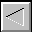|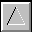|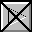|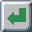|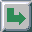TOC -- / --.-- / --.--.-- | Index | Search | Syntax | Help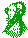-- Email comments, additions, corrections, gripes, kudos, etc. to:

Magnus Kempe -- Magnus.Kempe@di.epfl.ch